# Vector Algebra | Vector Diagram

Before studying electrical engineering it is essential to know that angular relation between mainly voltage and current in a system. For understanding the relation between voltage and we should first know definition of vector and go through vector algebra and vector diagram.

## Definition of Vector

There are some quantities which have both magnitude and as well as their direction of action. This type of quantities is called vector quantity. This is how one can make basic definition of vector in very few words. The most basic concept of a vector is that, it is a representation of these type of quantities in both magnitude and direction. Whenever we represent any quantity it may have some direction of action. Suppose if we say, a force of 5 N, it does not complete the picture.
We should always have to say the force in which direction, i.e. that 5 N force is in upward, downward or in any other directions. So the vector quantity must be represented with the magnetite as well as its direction. The direction of any quantity can be represented by measuring the angle formed by the direction of the quantity and a reference axis.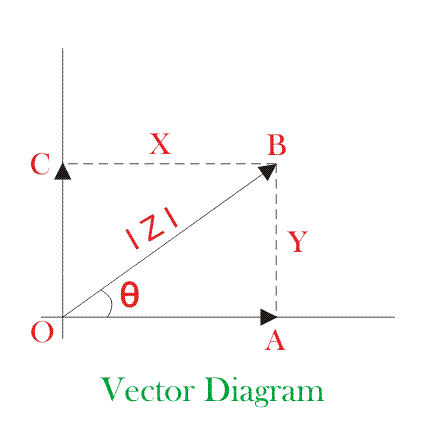Here in this vector diagram the vector OB has a magnitude of |Z| at an angle θ with reference axis ox. This may be resolved into two components at right angle to each other, say these are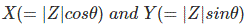The conventional method of representing vector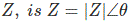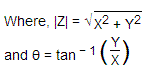## Vector Algebra

Now we will discuss about vector algebra. For different calcuilation, vector must be expressed algebraically. In the vector diagram the vector Z is the resultant of vectorically adding its components X and Y.
This vector may be written in vector algebra as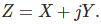Where, j indicates that the component Y is in perpendicular to component X. The x axis in the vector diagram is known as ‘real’ or ‘in-phase’ axis and the vertical y axis is called ‘imaginary’ or ‘quadrature’ axis. The symbol ‘j’ which is associated with the quadrature component Y, may be considered as an operator which rotates a vector anticlockwise through 90o. If a vector has to be rotated anticlockwise through 180o then the operator j has to perform its function twice and since the vector has reversed its sense then j.j or j2 = − 1

 Which implies, j = √ − 1

So we have seen that a vector quantity can be represented in following different forms,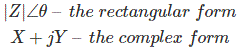#### Relation between rectangular and complex form of a vector

As per the vector diagram shown on this page. The magnitude of vector Z is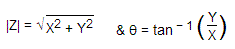From these two equations, we get,Putting these values of X and Y, in complex form of Z, we get,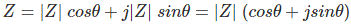The value of above expression is known as trigonometrical form of vector. Again we know that, cosθ and sinθ can be represented in exponential form as followsIf we put these above exponential form of sinθ and cosθ in the equation Z = |Z|(cosθ + jsinθ) we get,⇒ Z = |Z|e
This is the exponential form of vector.
Therefore from all above expressions of vector algebra and vector diagrams, it can be concluded that a vector quantity can be represented as total four basic form as listed below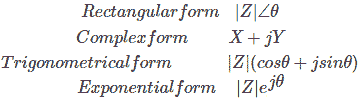Want To Learn Faster? 🎓
Get electrical articles delivered to your inbox every week.
No credit card required—it’s 100% free.